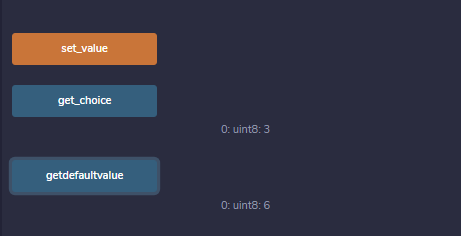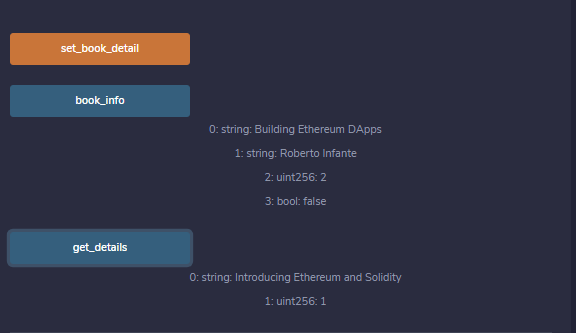Related Articles
• Solidity Tutorial

# Solidity – Enums and Structs

Enums are the way of creating user-defined data types, it is usually used to provide names for integral constants which makes the contract better for maintenance and reading. Enums restrict the variable with one of a few predefined values, these values of the enumerated list are called enums. Options are represented with integer values starting from zero, a default value can also be given for the enum. By using enums it is possible to reduce the bugs in the code.

Syntax:

```enum <enumerator_name> {
element 1, element 2,....,element n
} ```

Example: In the below example, the contract Types consist of an enumerator week_days, and functions are defined to set and get the value of a variable of the type enumerator.

## Solidity

 `// Solidity program to demonstrate ` `// how to use 'enumerator'` `pragma solidity ^0.5.0; ` ` `  `// Creating a contract` `contract` `Types {  `   `    ``// Creating an enumerator` `    ``enum` `week_days ` `    ``{ ` `      ``Monday, ` `      ``Tuesday, ` `      ``Wednesday, ` `      ``Thursday, ` `      ``Friday, ` `      ``Saturday, ` `      ``Sunday ` `     ``}  `   `    ``// Declaring variables of ` `    ``// type enumerator` `    ``week_days week;    ` `    `  `    ``week_days choice;`   `    ``// Setting a default value` `    ``week_days constant default_value ` `      ``= week_days.Sunday;` `    `  `    ``// Defining a function to ` `    ``// set value of choice` `    ``function set_value() ``public` `{` `      ``choice = week_days.Thursday;` `    ``}`   `    ``// Defining a function to ` `    ``// return value of choice ` `    ``function get_choice(` `    ``) ``public` `view returns (week_days) {` `      ``return` `choice;` `    ``}` `      `  `    ``// Defining function to` `    ``//  return default value` `    ``function getdefaultvalue(` `    ``) ``public` `pure returns(week_days) {  ` `        ``return` `default_value;  ` `    ``}  ` `}`

Output :### Struct

Structs in Solidity allows you to create more complicated data types that have multiple properties. You can define your own type by creating a struct.

They are useful for grouping together related data.

Structs can be declared outside of a contract and imported in another contract. Generally, it is used to represent a record. To define a structure struct keyword is used, which creates a new data type.

Syntax:

```struct <structure_name> {
<data type> variable_1;
<data type> variable_2;
}```

For accessing any element of the structure, ‘dot operator’ is used, which separates the struct variable and the element we wish to access. To define the variable of structure data type structure name is used.

Example: In the below example, the contract Test consists of a structure Book, and functions are defined to set and get values of the elements of the structure.

## Solidity

 `// Solidity program to demonstrate ` `// how to use 'structures'` `pragma solidity ^0.5.0;`   `// Creating a contract` `contract` `test {`   `   ``// Declaring a structure` `   ``struct` `Book { ` `      ``string name;` `      ``string writter;` `      ``uint id;` `      ``bool` `available;` `   ``}`   `   ``// Declaring a structure object` `   ``Book book1;`   `   ``// Assigning values to the fields ` `   ``// for the structure object book2` `   ``Book book2 ` `     ``= Book(``"Building Ethereum DApps"``, ` `            ``"Roberto Infante "``, ` `             ``2, ``false``);`   `   ``// Defining a function to set values ` `   ``// for the fields for structure book1` `   ``function set_book_detail() ``public` `{` `      ``book1 = Book(``"Introducing Ethereum and Solidity"``, ` `                   ``"Chris Dannen"``, ` `                    ``1, ``true``);` `   ``}`     `   ``// Defining function to print ` `   ``// book2 details` `   ``function book_info(` `   ``)``public` `view returns (` `     ``string memory, string memory, uint, ``bool``) {  ` `          `  `        ``return``(book2.name, book2.writter, ` `               ``book2.id, book2.available);  ` `    ``} ` `   `  `   ``// Defining function to print ` `   ``// book1 details` `   ``function get_details(` `   ``) ``public` `view returns (string memory, uint) {` `      ``return` `(book1.name, book1.id);` `   ``}` `}`

Output :